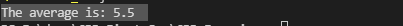Write a program to declare and initialize an array with 10 values (means give the values to array elements within the code, no need to take input from the user) and then display the average of the values of that array.

Sample Program:

The average is: 5.5

#include <iostream>
using namespace std;

void Arrays()
{
int arr = {1,2,3,4,5,6,7,8,9,10};
float sum = 0;
for (int i = 0; i < 10; i++)
{
sum += arr[i];
}
cout<<"The average is: "<<sum/10;
}
int main()
{
Arrays();
return 0;
}cpp programming exercise with solution